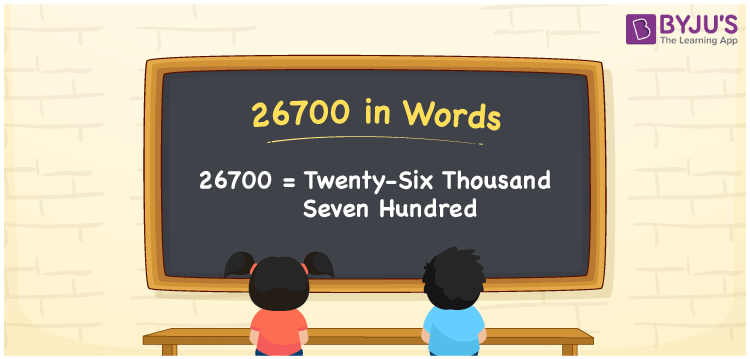# 26700 in Words

26700 in words is given by Twenty-Six Thousand Seven Hundred. For instance, Rohan purchased furniture for Rs. 26700, then you can say, “Rohan purchased furniture for Rupees Twenty-Six Thousand Seven Hundred”. The place value table is used to create the number name for 26700 in an easy way. 26700 is a cardinal number as it shows a specific amount. Let us understand the procedure of writing the number name for 26700 in detail here.

 26700 in Words Twenty-Six Thousand Seven Hundred Twenty-Six Thousand Seven Hundred in numerical form 26700

## 26700 in English Words

Usually, we write the numbers in words using the letters of the English alphabet. Therefore, we can read the number 26700 in English as Twenty-Six Thousand Seven Hundred.## How to Write 26700 in Words?

We use a place value system to convert the number 26700 into words easily. The place value chart for 26700 along with its expanded form is provided below.

 Ten Thousands Thousands Hundreds Tens Ones 2 6 7 0 0

Hence, we can write the expanded form as:

2 x Ten Thousand + 6 x Thousand + 7 x Hundred + 0 x Ten + 0 x One

= 2 x 10000 + 6 x 1000 + 7 x 100 + 0 x 10 + 0 x 1

= 20000 + 6000 + 700 + 0 + 0

= 20000 + 6000 + 700

= 26700

= Twenty-Six Thousand Seven Hundred

Therefore, 26700 in words is written as Twenty-Six Thousand Seven Hundred

Interesting way of writing 26700 in words

2 = Two

26 = Twenty-Six

267 = Two Hundred and Sixty-Seven

2670 = Two Thousand Six Hundred Seventy

26700 = Twenty-Six Thousand Seven Hundred

Thus, the word form of the number 26700 is Twenty-Six Thousand Seven Hundred

26700 is a natural number that precedes 26701 and succeeds 26699

• 26700 in words – Twenty-Six Thousand Seven Hundred
• Is 26700 an odd number? – No
• Is 26700 an even number? – Yes
• Is 26700 a perfect square number? – No
• Is 26700 a perfect cube number? – No
• Is 26700 a prime number? – No
• Is 26700 a composite number? – Yes

## Frequently Asked Questions on 26700 in Words

Q1

### Write 26700 in words.

26700 in words is written as Twenty-Six Thousand Seven Hundred.
Q2

### Simplify 20000 + 6700, and express in words.

Simplifying 20000 + 6700, we get 26700. Hence, the number 26700 in words is Twenty-Six Thousand Seven Hundred.
Q3

### 26700 is a prime number. True or False.

False, the number 26700 is not a prime number.